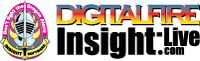•The secret to cool bodies and glazes is a lot of testing.
•The secret to know what to test is material and chemistry knowledge.
•The secret to learning from testing is documentation.
•The place to test, do the chemistry and document is an account at https://insight-live.com
•The place to get the knowledge is https://digitalfire.com

Sign-up at https://insight-live.com today.

# Sericite

 Oxide Analysis Formula CaO 0.22% 0.013 MgO 0.31% 0.026 K2O 6.88% 0.251 Na2O 0.10% 0.006 TiO2 0.22% 0.009 Al2O3 29.72% 1.000 SiO2 57.08% 3.260 Fe2O3 0.10% 0.002 LOI 4.65 Oxide Weight 324.77 Formula Weight 340.61

## XML for Import into INSIGHT

<?xml version="1.0" encoding="UTF-8"?> <material name="Sericite" descrip="" searchkey="" loi="0.00" casnumber=""> <oxides> <oxide symbol="CaO" name="Calcium Oxide, Calcia" status="" percent="0.220" tolerance=""/> <oxide symbol="MgO" name="Magnesium Oxide, Magnesia" status="" percent="0.310" tolerance=""/> <oxide symbol="K2O" name="Potassium Oxide" status="" percent="6.880" tolerance=""/> <oxide symbol="Na2O" name="Sodium Oxide, Soda" status="" percent="0.100" tolerance=""/> <oxide symbol="TiO2" name="Titanium Dioxide, Titania" status="" percent="0.220" tolerance=""/> <oxide symbol="Al2O3" name="Aluminum Oxide, Alumina" status="" percent="29.720" tolerance=""/> <oxide symbol="SiO2" name="Silicon Dioxide, Silica" status="" percent="57.080" tolerance=""/> <oxide symbol="Fe2O3" name="Iron Oxide, Ferric Oxide" status="" percent="0.100" tolerance=""/> </oxides> <volatiles> <volatile symbol="LOI" name="Loss on Ignition" percent="4.650" tolerance=""/> </volatiles> </material>